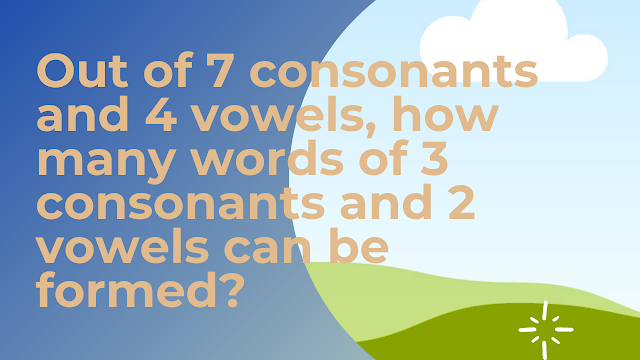Friday, February 3, 2023
HomeGeneral AptitudeOut of 7 consonants and 4 vowels, how many words of 3...

# Out of 7 consonants and 4 vowels, how many words of 3 consonants and 2 vowels can be formed?

##Question:

Out of 7 consonants and 4 vowels, how many words of 3 consonants and 2 vowels can be formed?

A. 25200               B. 21300

C. 24400               D. 210

3 consonants can be selected from 7 consonants in $^7C_3$ ways.

2 vowels can be selected from 4 vowels in $^4C_2$ ways.

$therefore$ by multiplication principle,
the number of selecting 3 consonants and 2 vowels is
$=^7C_3 times ^4C_2$

$=frac{7!}{3!4!} times frac{4!}{2!2!}$

$=frac{7.6.5}{3.2.1} times frac{4.3}{2.1}$

$=35 times 6$

$=210$

Now, the number of ways of arranging 5 letters among themselves
$=5!$
$=120$

$therefore$ the total number of words of 3 consonants and 2 vowels
$=210 times 120$
$=25200$
RELATED ARTICLES

### Dimension theorem of a quotient space: If W be a subspace of a finite dimensional vector space V over ​( mathbb{R} )​ then ​(...

$${}$$